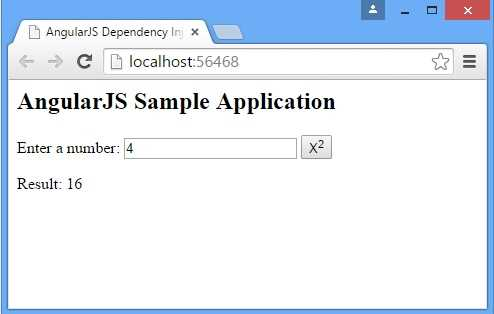# AngularJs Dependency Injection

Hi everyone in this blog I’m explaining about AngularJs Dependency Injection

##### Introduction:

Dependency Injection is a software design pattern in which components are given their dependencies instead of hardcoding them within the component. This relieves a component from locating the dependency and makes dependencies configurable. This helps in making components reusable, maintainable and testable.

AngularJS provides a supreme Dependency Injection mechanism. It provides following core components which can be injected into each other as dependencies.

• v  value
• v  factory
• v  service
• v  provider
• v  constant
##### Value:

Value is a simple JavaScript object and it is used to pass values to the controller during config phase.

`//define a modulevar mainApp = angular.module("mainApp", []);//create a value object as "defaultInput" and pass it a data.mainApp.value("defaultInput", 5);...//inject the value in the controller using its name "defaultInput"mainApp.controller('CalcController', function(\$scope, CalcService, defaultInput) {     \$scope.number = defaultInput;     \$scope.result = CalcService.square(\$scope.number);      \$scope.square = function() {         \$scope.result = CalcService.square(\$scope.number);     }});`
##### Factory:

The factory is a function which is used to return value. It creates value on demand whenever a service or controller requires. It normally uses a factory function to calculate and return the value.

`//define a modulevar mainApp = angular.module("mainApp", []);//create a factory "MathService" which provides a method multiply to return multiplication of two numbersmainApp.factory('MathService', function() {          var factory = {};       factory.multiply = function(a, b) {         return a * b      }    return factory;});  //inject the factory "MathService" in a service to utilize the multiply method of factory.mainApp.service('CalcService', function(MathService){    this.square = function(a) {         return MathService.multiply(a,a);     }});`
##### Service:

Service is a singleton JavaScript object containing a set of functions to perform certain tasks. Services are defined using service () functions and then injected into controllers.

`//define a module    var mainApp = angular.module("mainApp", []);    ...    //create a service which defines a method square to return square of a number.    mainApp.service('CalcService', function(MathService){        this.square = function(a) {             return MathService.multiply(a,a);         }    });    //inject the service "CalcService" into the controller    mainApp.controller('CalcController', function(\$scope, CalcService, defaultInput) {        \$scope.number = defaultInput;        \$scope.result = CalcService.square(\$scope.number);         \$scope.square = function() {            \$scope.result = CalcService.square(\$scope.number);        }    });`
##### Provider:

Provider is used by AngularJS internally to create services, factory etc. during config phase(phase during which AngularJS bootstraps itself). Below mention script can be used to create Math Service that we've created earlier. Provider is a special factory method with a method get () which is used to return the value/service/factory.

`//define a module    var mainApp = angular.module("mainApp", []);    ...    //create a service using provider which defines a method square to return square of a number.    mainApp.config(function(\$provide) {        \$provide.provider('MathService', function() {            this.\$get = function() {                var factory = {};                  factory.multiply = function(a, b) {                    return a * b;                 }                return factory;            };        });    });`
##### Constant:

constants are used to pass values at config phase considering the fact that value can not be used to be passed during config phase.

mainApp.constant("configParam", "constant value");

#### Example:

Following example will showcase all the above mentioned directives.

`<html><head>    <title>AngularJS Dependency Injection</title></head><body>    <h2>AngularJS Sample Application</h2>    <div ng-app="mainApp" ng-controller="CalcController">        <p>            Enter a number:            <input type="number" ng-model="number" />            <button ng-click="square()">X<sup>2</sup></button>        <p>Result: {{result}}</p>    </div>    <script src="http://ajax.googleapis.com/ajax/libs/angularjs/1.3.14/angular.min.js"></script>    <script>        var mainApp = angular.module("mainApp", []);         mainApp.config(function (\$provide) {            \$provide.provider('MathService', function () {                this.\$get = function () {                    var factory = {};                    factory.multiply = function (a, b) {                        return a * b;                    }                    return factory;                };            });        });         mainApp.value("defaultInput", 4);         mainApp.factory('MathService', function () {            var factory = {};            factory.multiply = function (a, b) {                return a * b;            }            return factory;        });         mainApp.service('CalcService', function (MathService) {            this.square = function (a) {                return MathService.multiply(a, a);            }        });         mainApp.controller('CalcController', function (\$scope, CalcService, defaultInput) {            \$scope.number = defaultInput;            \$scope.result = CalcService.square(\$scope.number);             \$scope.square = function () {                \$scope.result = CalcService.square(\$scope.number);            }        });    </script></body></html>`

##### Output:Last updated:2/22/2018 3:16:13 AM#### Anonymous User

I am a content writter !